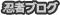×

[PR]上記の広告は3ヶ月以上新規記事投稿のないブログに表示されています。新しい記事を書く事で広告が消えます。

／∥￣∥￣∥＼
Γ￣￣￣￣￣￣￣￣￣||
|　　　FRONT　　　　||
|　　　 　　　　　　||
|　TOWARD　ENEMY　　||
Ｌ＿＿＿＿＿＿＿＿＿||
∧　　　　∧
PR
［　］
＼〇＼
∥
Г∟
ここに一つあって、

それをすり減らして毎日生きてる。多分。

いろいろな、

いまは、まぁるくてやらかいもの。

ご存知ありませんか？

3.3 Answer)

a) Let kinetic energy increased be equal to k

k = 1/2m(v22-v11) = 216000 = 216 kJ/kg

b) Let the change in enthalpy be equal to Δh

Δh = {(u2-u1)+(p2v2-p1v1)}×103 =  {(125-292)+(40×1/0.8-700×1/6.0)}103
= -234.666...×103 = -234 kJ/kg

c) Change in flow energy (i.e, pV-work) f is,

f = p2v2-p1v1 = -66.66666 = -66.7 kJ/kg

d) Let the changes in potential energy per unit of mass be equal to u. u is given by,

u = g (h2-h1) = 98 J/kg

e) The mass flow rate per 1 sec. is,

R = (0.15/2)2×π×60×6.0 = 6.3617.... =6.4kg/s

(cf. (0.124/2)2×π×660×0.8 = 6.3762....)

f) The changes in total energy per 1 sec. between point (1) and (2) is,

ΔE = R(k+u+Δh) = -144572 = -115 kW

e) From the answer of question (f), the flow gas loses 115kJ/s between (1) and (2),
in the other words, the gas works 115kW on the surroundings except heat gained.
Thus, the power transferred to the surroundings between (1) and (2) is given by
following equation.

W = 50×6.4 + 115 = 435 kW

h) From the relation between specific heats and gas constant of ideal gas, we get,

Cp-Cv = R
Cp = R + Cv
∴Cp = 280 + 700 = 980 J/(kg・K)

3.1 Answer)

Total energy Et=1/2mv2+mgz+U+pV

Potential energy charges are negligible, so we get,
Et = 1/2mv2+H (H - Enthalpy)

Entering energy per 1 second is given by following equation
E1=1/2mv12+H1

The energy theta exiting flow has is given by following equation
E2=1/2mv22+H2

We can neglect the heat loss, we get the power generated per 1 sec. by following equation

P[J/s]   = E1 - E2 = 1.8［1/2(202-382)+(3140-2500)×103］=1151060.4 = 1151 kW

Calendar.....07 2019/08 09
S M T W T F S
1 2 3
4 5 6 7 8 9 10
11 12 13 14 15 16 17
18 19 20 21 22 23 24
25 26 27 28 29 30 31
Uniqlock.....
Category.....
Lastest Article.....
(08/16)
(10/17)
(10/16)
(08/17)
(07/25)
Profile.....
Iiyamaline

Mobile Accsess.....
Article Search.....
Archive.....